# Expression 6

Evaluate expression: -6-2(4-8)-9

x =  -7

### Step-by-step explanation: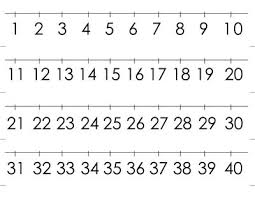Did you find an error or inaccuracy? Feel free to write us. Thank you!## Related math problems and questions:

• Expression 8Evaluate this expressions: a) 5[3 + 4(2.8 - 3)] b) 5×(8-4)÷4-2
• Evaluate - order of opsEvaluate the expression: 32+2[5×(24-6)]-48÷24 Pay attention to the order of operation including integers
• Evaluate expressionIf x=2, y=-5 and z=3 what is the value of x-2y
• With bracket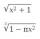Calculate (evaluate) simple mathematical expression with a negative numbers and a bracket: 13+15*5-2*(-6)
• Expression plus minusEvaluate expression: (-1)2 . 12 – 6 : 3 + (-3) . (-2) + 22 – (-3) . 2
• Expression 1What is 7+8-(5×2)+5-4+(6×(5-3)+6)-(8+10)-7+6?
• Evaluate expression 2Evaluate expression with negatives: (-3)+4+(-8)+(-6)+4+(-1)Why does 1 3/4 + 2 9/10 equal 4.65? How do you solve this?
• Integers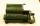May be the sum of two integers less than their difference?Add marks (+, -, *, /, brackets) to fullfill equations 1 3 6 5 = 10 This is for the 4th grade of the primary school - with no negative numbers yet
• Evaluate 17Evaluate 2x+6y when x=- 4/5 and y=1/3. Write your answer as a fraction or mixed number in simplest form.
• Product of the sum and differenceCalculate the product of the sum and difference of numbers -7 and -2.
• Brackets 2Add parenthesis to make true: 5-2×6-4+2=5Add two mixed fractions: 2 4/6 + 1 3/6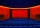All pupils from the school went to the cinema for a film performance. The cinema has 400 seats, 2 rows of 20 seats remain unoccupied. Teachers occupied 10 places. How many students were there?Add this two mixed numbers: 1 5/6 + 2 2/11=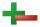Calculate opposite numbers (additive inverse) to given ones: